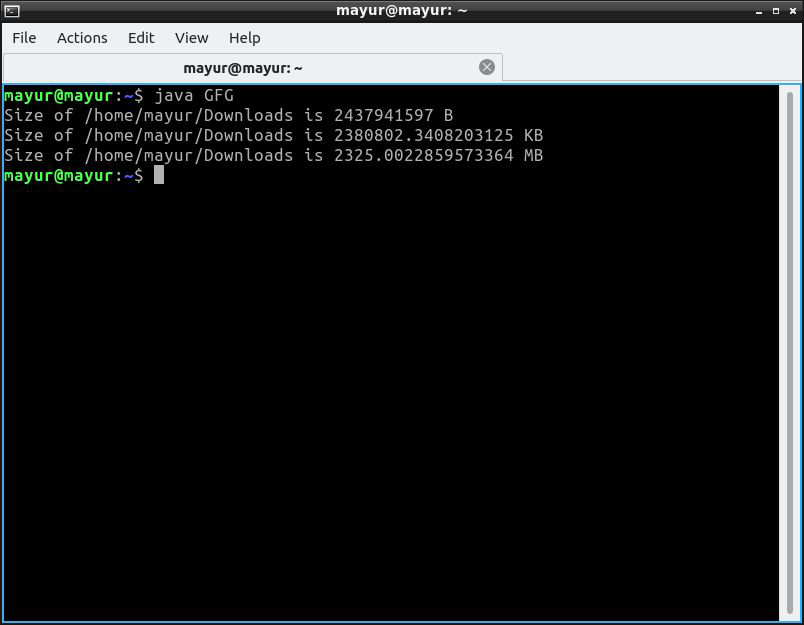# Java Program to Get the Size of a Directory

• Last Updated : 27 Apr, 2021

The size of files in Java can be obtained using the File class. The in-built function of ‘fileName.length()’ is used to find size of file in Bytes. The directory may contain ‘N’ number of files, for calculating the size of the directory summation of the size of all the files is required.

length() Method:

length() method in the object of the file class is returning the file size in long(datatype) format. The file size is in a unit of Byte.

Return Type: long

Syntax:

```java.io.File file = new java.io.File("file_name.txt");
file.length();```

Working:

1. If the file exists then the function return the size of the file
2. Else the function returns null.

However, the size of the file can be display in Mega, Kilo units using unit conversion.

## Java

 `// Java program to Get the size of a directory` `import` `java.io.File;` `class` `GFG {``    ``private` `static` `long` `getFolderSize(File folder)``    ``{``        ``long` `length = ``0``;``      ` `        ``// listFiles() is used to list the``        ``// contents of the given folder``        ``File[] files = folder.listFiles();` `        ``int` `count = files.length;` `        ``// loop for traversing the directory``        ``for` `(``int` `i = ``0``; i < count; i++) {``            ``if` `(files[i].isFile()) {``                ``length += files[i].length();``            ``}``            ``else` `{``                ``length += getFolderSize(files[i]);``            ``}``        ``}``        ``return` `length;``    ``}` `    ``// Driver Code``    ``public` `static` `void` `main(String[] args)``    ``{``        ``// Creating an instances of file class``        ``File file1 = ``new` `File(``"/home/mayur/Downloads"``);` `        ``long` `size = getFolderSize(file1);``        ``// Size of folder in Bytes``        ``System.out.println(``"Size of "` `+ file1 + ``" is "``                           ``+ size + ``" B"``);``        ``// Size of folder in Kilobytes``        ``System.out.println(``"Size of "` `+ file1 + ``" is "``                           ``+ (``double``)size / ``1024` `+ ``" KB"``);``        ``// Size of folder in Megabytes``        ``System.out.println(``"Size of "` `+ file1 + ``" is "``                           ``+ (``double``)size / (``1024` `* ``1024``)``                           ``+ ``" MB"``);``    ``}``}`

#### Output:My Personal Notes arrow_drop_up